# Pearl No.4 - Kth Smallest in the Union of 2 Sorted CollectionsHere is the Pearl No.4:

Let A and B be two disjoint ordered collections with distinct elements inside. Their combined size is greater than k.

1. A and B are sorted, but the underlying data structures are not specified as they are collections.
2. A and B do not have common elements, since they are disjoint
3. All elements in A and B are distinct
4. The total number of all elements is larger than k

Find the kth smallest element of A ∪ B.

By definition, the kth smallest element of a collection is one for which there are exactly k elements smaller than it, so the 0th smallest is the smallest, i.e., k starts from 0.

Let's have a look at an example.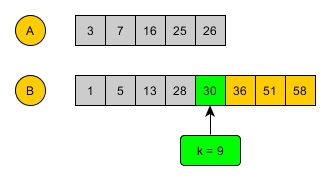# Easy Solution - Merge

I believe it is easy enough to come out with the merge solution. Since both A and B are sorted, we can just start from the beginning of A and B and do the regular merge operation until we reach kth. (In fact, when I tried to produce the example figure above with k = 7, I did use merge in my head to find the target element 36.)

Say A and B are lists, we can have the following solution in OCaml:

let kth_merge a b k =
let rec merge i = function
| x::_, y::_ when i = k -> min x y
| m::_, [] | [], m::_ when i = k -> m
| _::ms, [] | [], _::ms when i < k -> merge (i+1) ([], ms)
| x::xs, y::ys when i < k ->
merge (i+1) (if x < y then xs, y::ys else x::xs, ys)
| _ -> assert false
in
merge 0 (a,b)


The time complexity of this is obviously O(n) or more precisely O(k).

This approach assumes A and B being lists and merge should be a quite optimal solution. However, the problem doesn't force us to use list only. If we assume A and B to be array, can we do better?

# Recall Binary Search

As described in Mutable, when looking for an element in a sorted array, we can use binary search to obtain O(log(n)) performance, instead of linear searching.

For binary search, we just go to the middle and then turn left or right depending on the comparison of the middle value and our target.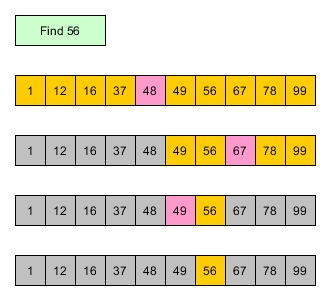Coming back to our current problem,

1. We are searching for something (a value with a rank instead of a particular value itself though)
2. The data structures of A and B are not limited to lists, but also can be arrays.
3. Both A and B are sorted.

These characteristics present nothing directly towards a classic binary search problem, however, they seem hinting us that binary search is worth trying.

Let's have a go.

# Double Binary Rank Search

So here is the A and B, we want to find the kth smallest value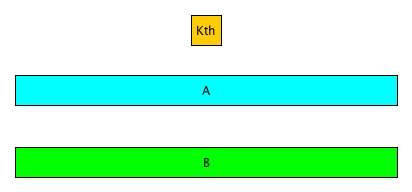Since we are trying binary search, we can just split A and B by their middle values respectively.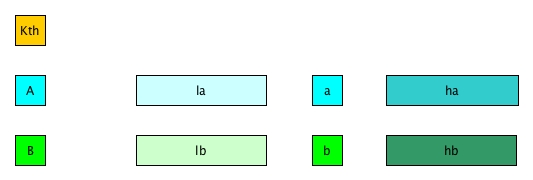So what now?

In single binary search, we can compare the middle value with the target varlue. And if target is larger, then we go right; otherwise, we go left.

But in this double binary rank search problem, it is a little trickier:

1. We have 2 sorted arrays instead of 1
2. We are not searching for an element with a particular value, instead, with a particular rank (kth smallest)

From point 1 above, the middle values split the two arrays into four parts; From point 2 above, we have to find out which part (out of the four) the kth would fall into. In addition, since its about rank instead of a value, it must be related to the lengths of the parts.

Let's assume a < b (if not, then we can simply swap A and B).

So if a < b, what we can induct?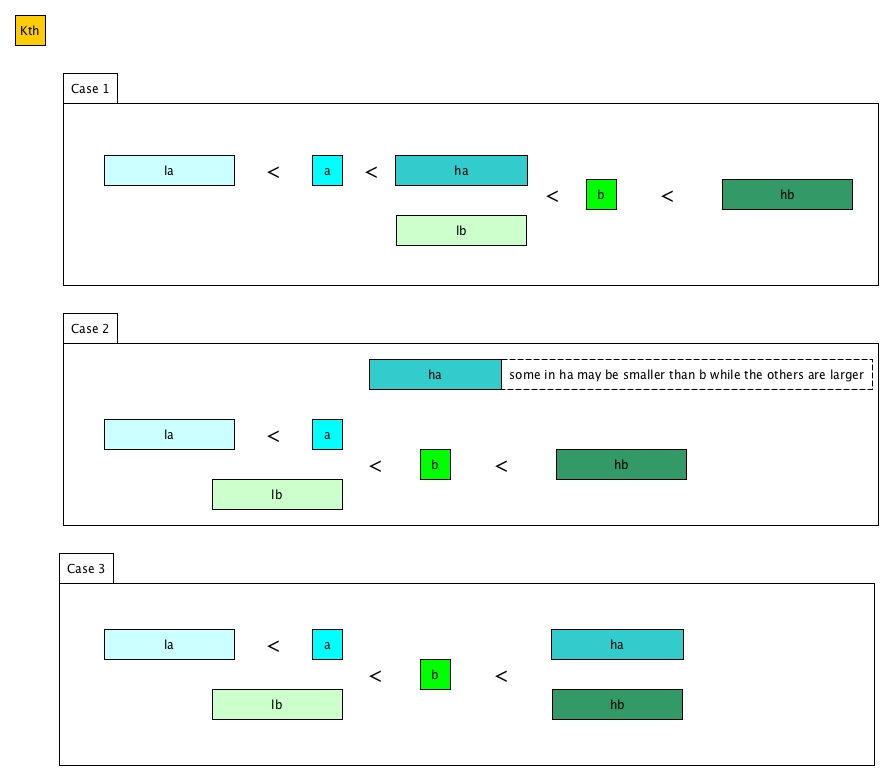The relationships between all the parts (including a and b) are demonstrated as above. The position of ha is dynamic as we only know the middle points a < b. However, the relationship between la, a, lb and b is determined and we know for sure that there are definitely at least la + lb + 1 (lenth of a is 1) numbers smaller than b. Thus considering the rank k, if k <= la + lb + 1:

1. From Case 1 and 2, the kth element must be in la, or a, or ha, or lb
2. From Case 3, it must be in la, or a, or lb

We therefore can know simply that if k <= la + lb + 1, the kth smallest number definitely won't be in hb.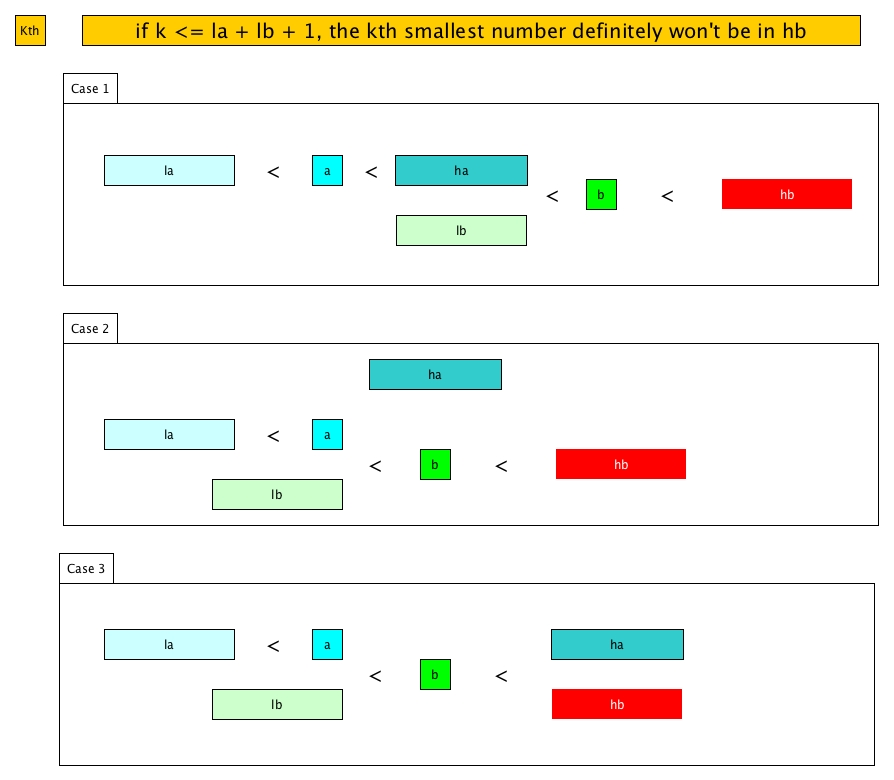What if k > la + lb + 1? Going back to the 3 cases:

1. The kth element might be in hb because k can be big enough.
2. From Case 1, it might be in ha if ha are all larger than lb otherwise, it might be in lb
3. From Case 2, it might be in ha or b
4. From Case 3, it is obviously that la doesn't have a chance to have the kth element.

Thus if k > la + lb + 1, the kth smallest number definitely won't be in la.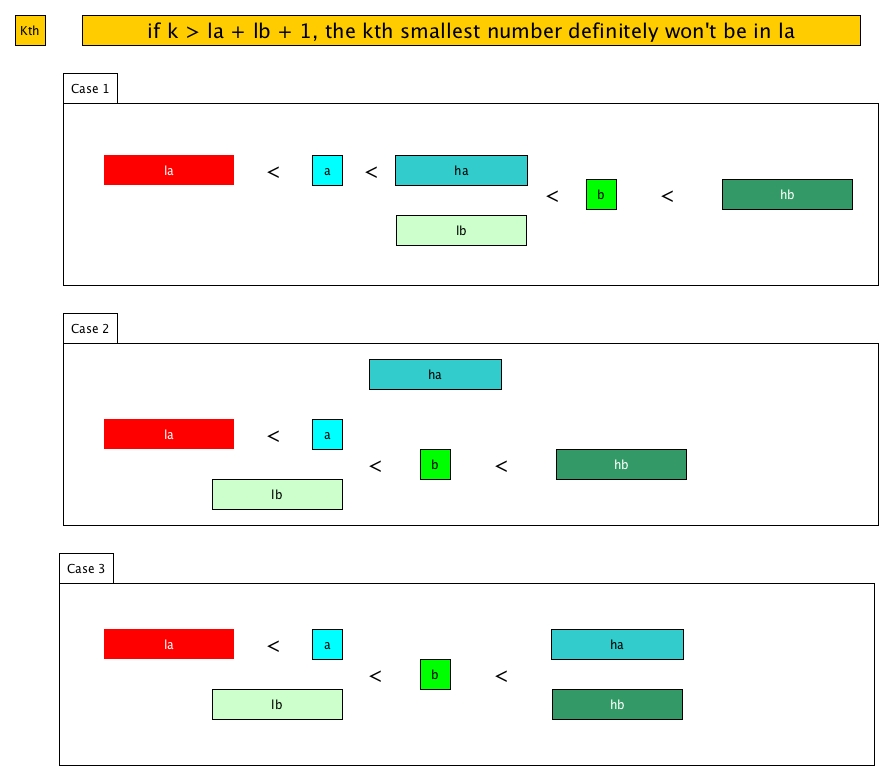So every time we compare k with la + lb + 1 and at least one part can be eliminated out. If a < b, then either la or hb is removed; otherwise, either lb or ha is removed (as said, we can simply swap A and B).

let kth_union k a b =
let sa = Array.length a and sb = Array.length b in
let rec kth k (a, la, ha) (b, lb, hb) =
if la >= ha+1 then b.(k+lb)
else if lb >= hb+1 then a.(k+la)
else
let ma = (ha+la)/2 and mb = (hb+lb)/2 in
match a.(ma) < b.(mb), k >= ma-la+1+mb-lb with
| true, true -> kth (k-(ma-la+1)) (a, ma+1, ha) (b, lb, hb)
| true, false -> kth k (a, la, ha) (b, lb, mb-1)
| _ -> kth k (b, lb, hb) (a, la, ha) (* swap *)
in
kth k (a, 0, sa-1) (b, 0, sb-1)


Since every time we remove 1 / 4 elements, the complexity is O(2*log(N)), i.e., O(log(N)).

# Remark

This pearl is comparatively not difficult, however, the idea of process of elimination is worth noting. Instead of choosing which part is positive towards our answer, we can remove parts that is impossible and eventually we can achieve similar algorithmic complexity.

# A further slight improvement

From a reply in hackernews, it was suggested that

If the data structures can be split (as it seems), then the first step should be to get the head k element of both collections, so we only have to work with a maximum of 2k elements. Then the solution is not O(2 * log(N)) but O(2 * log(min(N,2k))).

This would not significantly improve the performance however it makes sense especially when k is smaller than the lengths of both A and B.